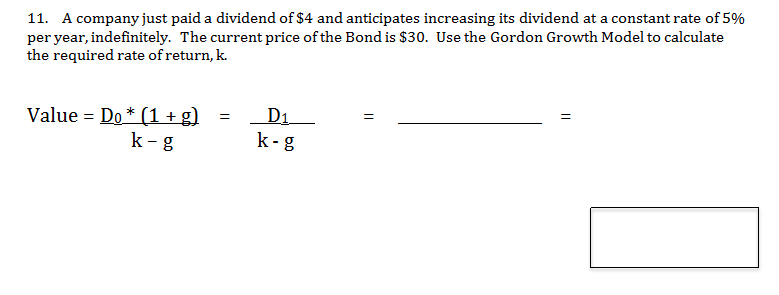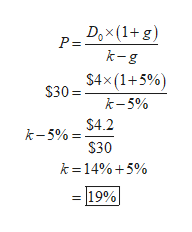# 11. A company just paid a dividend of $4 and anticipates increasing its dividend at a constant rate of 5%per year, indefinitely. The current price of the Bond is$30. Use the Gordon Growth Model to calculatethe required rate of return, k.Value Do (1+gk-gD1k-g

Questionhelp_outlineImage Transcriptionclose11. A company just paid a dividend of $4 and anticipates increasing its dividend at a constant rate of 5% per year, indefinitely. The current price of the Bond is$30. Use the Gordon Growth Model to calculate the required rate of return, k. Value Do (1+g k-g D1 k-g fullscreen
check_circle

Step 1

Calculate the required rate ...help_outlineImage TranscriptioncloseDox(1+g) P = k-g $4x(1+5%)$30= k-5% $4.2 k-5%$30 k =14%5% 19% fullscreen

### Want to see the full answer?

See Solution

#### Want to see this answer and more?

Solutions are written by subject experts who are available 24/7. Questions are typically answered within 1 hour.*

See Solution
*Response times may vary by subject and question.
Tagged in